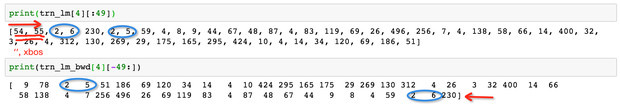# ULMFiT backwards models

The ULMFiT IMDB scripts allow one to use a backwards model.

However, I’m pretty dumb and can’t work how how the fine tuning or classification part actually passes the data backwards.

Superfically it looks like the LanguageModelLoader constructor can take a backwards parameter, but it isn’t being used, even though it does load the correct WikiText model.

How does this work?

@sebastianruder (you are going to regret asking to be tagged in questions about these scripts!)

1 Like

HahaThat’s a great point, though!

In our experiments, we used a separate script to create the training data for the backwards language model. I haven’t uploaded that script yet, as I thought it was quite ugly to create separate files. It’d be nicer to simply transform the data once if the `backwards` parameter is set. Thoughts?

I’m fine with the backwards parameter, but unclear if I should reverse the BOS and FLD annotations in files too? This part:

``````def get_texts(df, n_lbls=1):
labels = df.iloc[:,range(n_lbls)].values.astype(np.int64)
texts = f'\n{BOS} {FLD} 1 ' + df[n_lbls].astype(str)
for i in range(n_lbls+1, len(df.columns)): texts += f' {FLD} {i-n_lbls} ' + df[i].astype(str)
texts = texts.apply(fixup).values.astype(str)

tok = Tokenizer().proc_all_mp(partition_by_cores(texts))
``````

I also think I’m missing something - my classifier based on the backwards language model is about 8% worse in accuracy than my forward one. That seems wrong, but I can’t find the problem (and I’ve added the `backwards=True` parameter to the `TextDataset` parts.

Just to confirm, the backwards classifier should be roughly as strong as the forward one, right?

1 Like

So far, we basically kept the FLD annotations fixed so that the model still knows which field it is in and reverse only the text. For context, here’s the script we’ve been using to transform forward ids into backward ids.
Yep, the backwards and the forward model should have roughly similar performance.

``````import numpy as np
import fire
from create_toks import FLD
import pickle

def _partition_cols(a,idxs):
i=0
for idx in idxs:
yield a[i:i+idx]
i+=idx
yield a[i:]

def partition_cols(a,idxs): return list(_partition_cols(a,idxs))

def reverse_flds(t, fld_id):
t = np.array(t)
idxs = np.nonzero(t==fld_id)
parts = partition_cols(t,idxs)[1:]
reversed = np.concatenate([np.concatenate([o[:2],o[:1:-1]]) for o in parts[::-1]])
return reversed

def create_bw_data(prefix, joined=False):
print(f'prefix {prefix}; joined {joined}')
PATH=f'data/nlp_clas/{prefix}/'
joined_id = 'lm_' if joined else ''

fwd_trn_path = f'{PATH}tmp/trn_{joined_id}ids.npy'
fwd_val_path = f'{PATH}tmp/val_{joined_id}ids.npy'

bwd_trn_path = f'{PATH}tmp/trn_{joined_id}ids_bwd.npy'
bwd_val_path = f'{PATH}tmp/val_{joined_id}ids_bwd.npy'

stoi = {s: i for i, s in enumerate(itos)}
fld_id = stoi[FLD]

bwd_trn = np.array([reverse_flds(o, fld_id) for o in fwd_trn])
bwd_val = np.array([reverse_flds(o, fld_id) for o in fwd_val])

np.save(bwd_trn_path, bwd_trn)
np.save(bwd_val_path, bwd_val)

if __name__ == '__main__': fire.Fire(create_bw_data)
``````
2 Likes

Hi @sebastianruder
Thanks for your paper, I’m working on implementing ULMFit on our Chinese custom service corpus. I succeeded to train forwards model and now i am working on backwards models. When i try out your codes above, I found some problems. Is `_partition_cols` wrong, and I use the modified version as blew:

``````def _partition_cols(a,idxs):
i=0
for idx in idxs:
yield a[i:idx]
i=idx
yield a[i:]
``````

It works well: 2 is fld_id and (5,6) are fld_seq_idI have another question about “xbos”. Do we need to add “” and xbos at the head of each backwards rows as we did for forwards model?

1 Like

@jeremy could you give some idea about “xbos” in backwards models?

Yes you probably want `xbos` in the same spot for backwards. Try it!Thx, I am training the backwards LM model and get the similar result as forward model in the first epoch.
Forward
epoch || trn_loss || val_loss || accuracy
0 || 3.919522 || 3.161851 || 0.43043
backward:
epoch || trn_loss || val_loss || accuracy
0 || 3.934117 || 3.171467 || 0.428741

The issue with these tokens is really how they impact the classification model, so you should test that too.

Got it, I will try it out after i get the backward LM model.